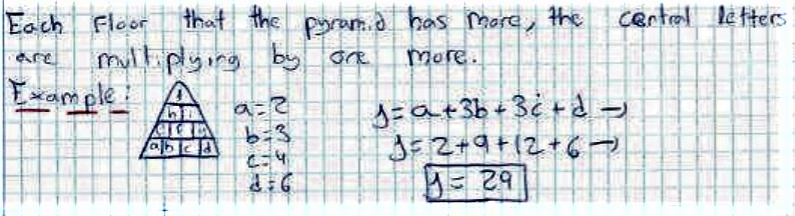#### You may also like### Summing Consecutive Numbers

15 = 7 + 8 and 10 = 1 + 2 + 3 + 4. Can you say which numbers can be expressed as the sum of two or more consecutive integers?### Always the Same

Arrange the numbers 1 to 16 into a 4 by 4 array. Choose a number. Cross out the numbers on the same row and column. Repeat this process. Add up you four numbers. Why do they always add up to 34?### Fibs

The well known Fibonacci sequence is 1 ,1, 2, 3, 5, 8, 13, 21.... How many Fibonacci sequences can you find containing the number 196 as one of the terms?

# Number Pyramids

##### Age 11 to 14Challenge Level

Can you work out how the numbers in the upper layers are generated?
Bela from QASMT and Nabiha and Shahzaib from Greenacre Public School in Australia, Muhammad from Walton High School in the UK, Shriya from International School Frankfurt in Germany and Philip and Ikhee from Dulwich College Seoul in South Korea worked this out. Muhammad sent an example: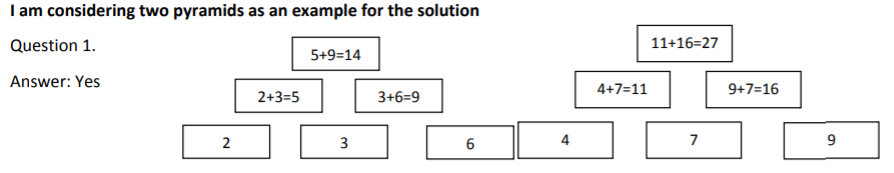Umar from Greenacre Public School, Daniele from Southlands International School in Italy, and Tuáº¥n Vé from British Vietnamese International School Hanoi in Vietnam used this method, described by Umar, to find the number at the top of the pyramid without finding the numbers in the middle:
To find the top numbers, you must [add] the edge numbers and add the product of the middle number and two.

Daniele explained the effect of changing the order of the numbers, and how to make the top of the pyramid as large as possible:
If I only swap the numbers at the sides in the bottom layer, the top number will stay the same. If I change the middle number then the top number will change.

Given any three numbers for the bottom, the largest possible top number can be found, placing the highest number of the three in the middle.

Anh Minh from British Vietnamese International School Hanoi, James, Minoo and Jay, Julie, Kristin, Lily and Ji Hyo from Dulwich College Seoul, Kelvin from QASMT, Rik from G.D.Goenka World School in India, Connor from Tanglin Trust School in Singapore, David from IES Maximo Laguna in Spain and Philip and Ikhee used algebra to find the top number without finding the middle numbers. This is Anh Minh's work:
Lets say this is the pyramid

F

D           E

A           B           C

So A + B = D,  B + C = E,  D + E = F

So D + E = A + B + B + C

So A + 2B + C = F

Kelvin, Julie, Kristin, Lily, Ji Hyo, Philip and Ikhee explained why the order is important. Kelvin wrote:
If we switch the numbers in the bottom row, $a$, $b$ and $c$ will be switched.
Because $a+2b+c \ne b+2a+c ...$ etc, the number at the top will change.

Julie, Kristin, Lily and Ji Hyo explained how to get the largest possible number at the top:
The number that is being added twice ($b$) should be the largest because despite the two edge numbers changing sides it won't make any difference to the value of the top number

If I give you a target for the top number, can you quickly find three possible numbers for the bottom?
Raffaele and Shriya worked backwards from the description of how to find the top number from the bottom numbers. This is Raffaele's work: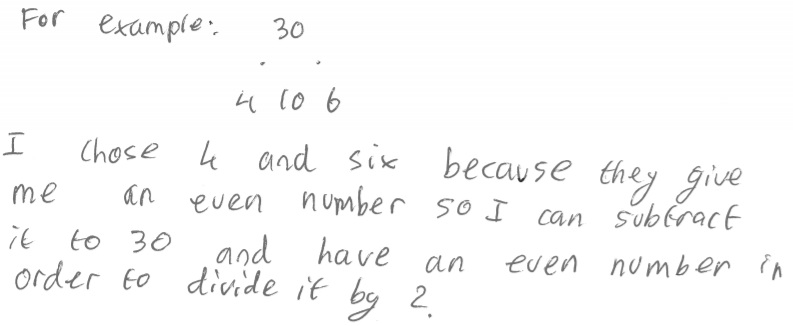Daniele used a similar method involving subtraction:
a) First find two possible numbers that add up to the top number (e.g. if the top number is 27 it could be 13 and 14).
b) Then find one number that can be subtracted from both numbers (e.g. 13$-$6 = 7 and 14$-$6 = 8)
c) The two result numbers obtained from the step above (e.g. 7 and 8) go on the sides of the bottom layer
d) The number used to subtract in sten b) goes in the middle.

Connor and Kelvin used their algebraic expressions. They both chose the numbers at the edges first, and then worked out the middle numbers.
Kelvin:
Let the top number be $y$.

The number on top is $a+2b+c.$ Because $2b$ is even,

If the top number is even, $a+c$ must be even, so let $a=1$, $c=1.$
Then, $b=(y-2)\div2= 0.5y-1.$

If $y$ is odd,
$a+c$ is odd, so let $a=1$, $c=2.$
Then, $b=(y-3)\div2=0.5y - 1.5$

Connor:
If $n$ is even:
$a=0$
$b=\frac{n}{2}$
$c=0$

If $n$ is odd:
$a=1$
$b=\frac{n+1}2$
$c=0$

Can you adapt what you discovered about 3-layer pyramids to larger pyramids?
James, Jay and Minoo showed how a 4-layer pyramid can be treated as two 3-layer pyramids: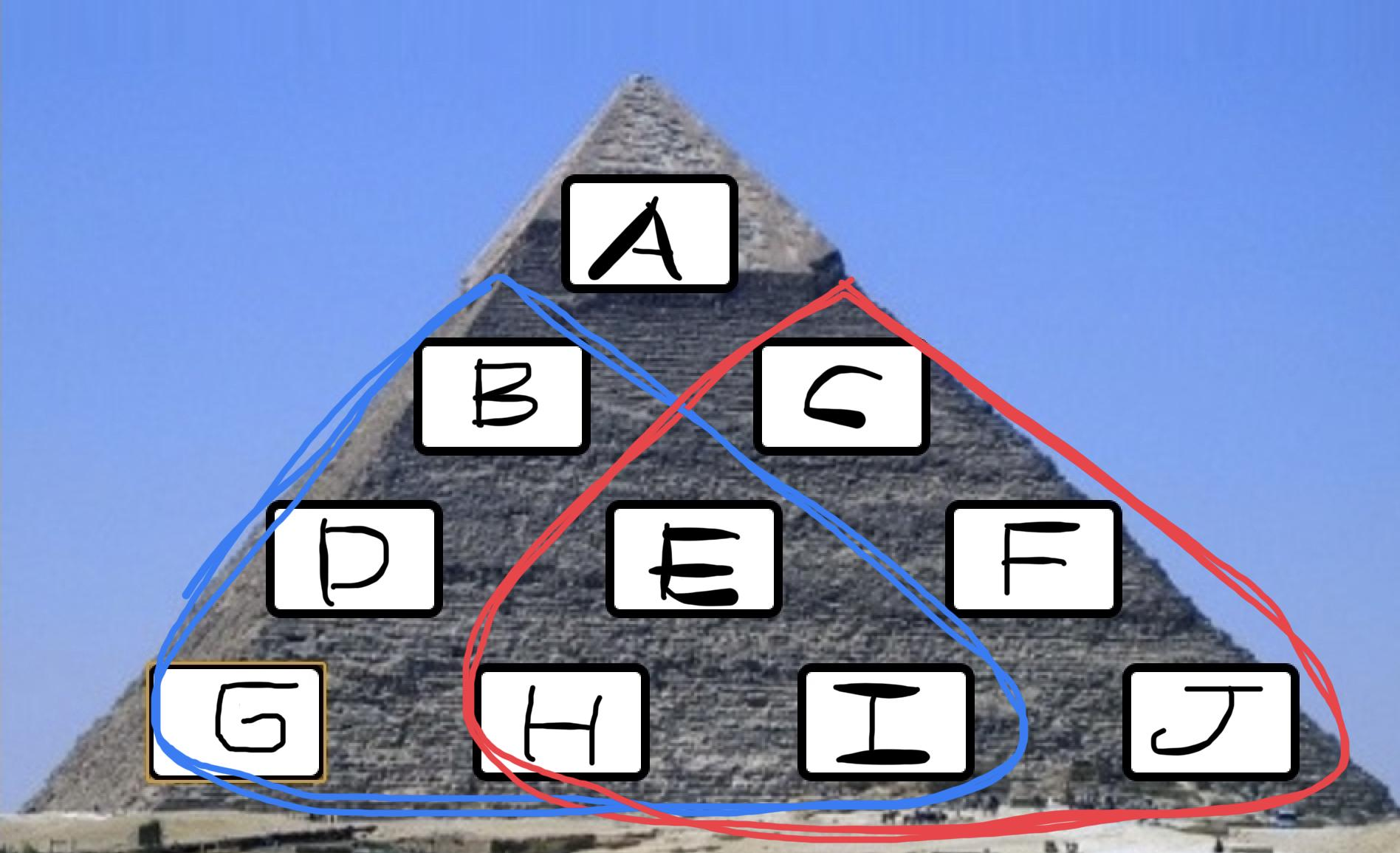You can use information from 3 layer pyramid.

There are two 3 layer pyramids in the 4 layer pyramid.

A  =  B +  C

B  =  G  +  H  +  H  +  I

C  =  H  +  I  +  I  +  J

A  =  B  +  C  =  G  +  H  +  H  +  I  +  H  +  I  +  I  +  J

David sent in a method to choose numbers at the bottom of a 4-layer pyramid to get a target number at the top: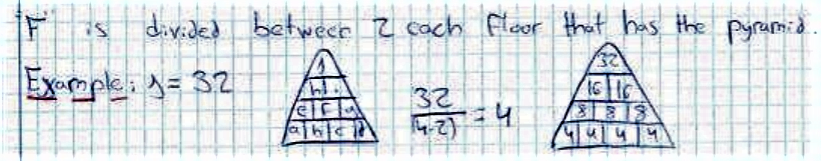Raffaele and David also used algebra again. This is David's work: